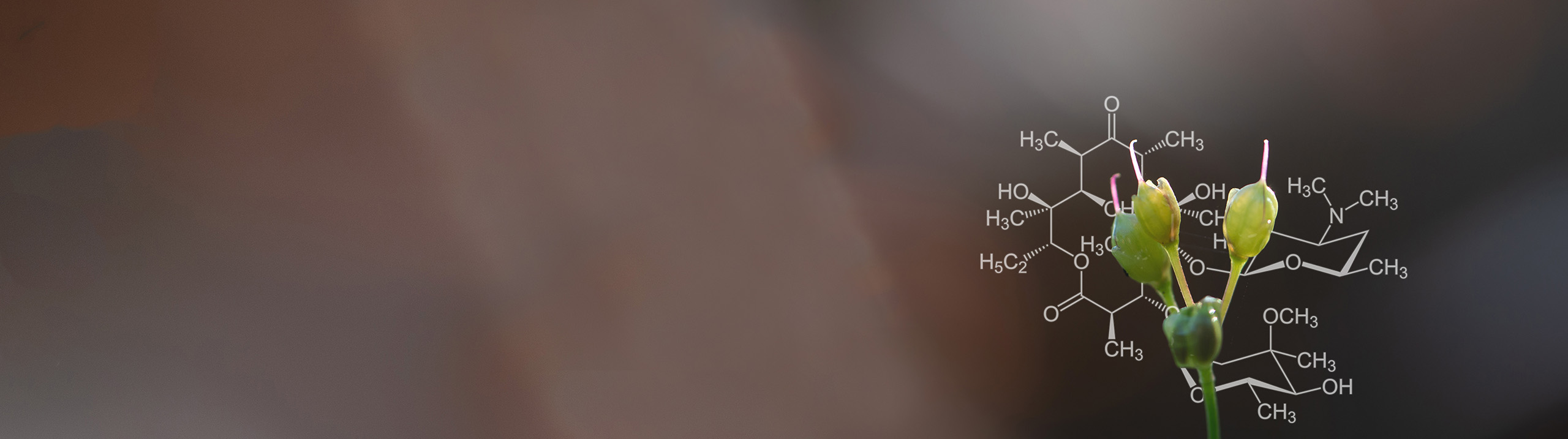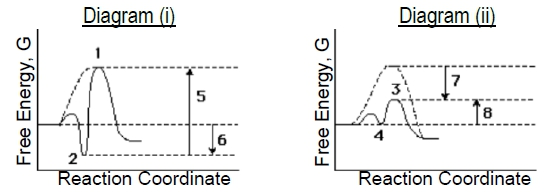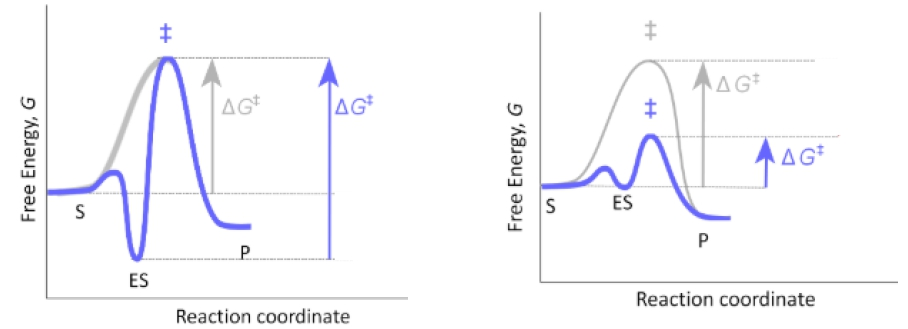Start typing, then use the up and down aroows to select an option from the list.# Biochemistry

Learn the toughest concepts covered in Biochemistry with step-by-step video tutorials and practice problems by world-class tutors

6. Enzymes and Enzyme Kinetics

# Lock and Key Vs. Induced Fit Models

Next Topic1

### concept

Lock-and-Key Vs. Induced Fit Models4m
Play a video:
0
2

### concept

Lock and Key Vs. Induced Fit Models4m
Play a video:
0
3
Problem

Compare the two enzyme-catalyzed reaction diagrams below (i & ii) to determine which of the following is true.a) The ES-complex in diagram (i) is #2 and in diagram (ii) is #3..

b) Catalyzed EA in diagram (i) is arrow #5 and in diagram (ii) is arrow #7.

c) Binding energy in diagram (i) is arrow #5 and in diagram (ii) is arrow #7.

d) Diagram (i) describes a “lock & key” model while (ii) describes more of an “induced fit” model.

4
Problem

What is a potential disadvantage for an enzyme having too high of an affinity for its substrate?

5
Problem

Select the best option that fills in the blanks appropriately in the order of their appearance.The left graph depicts an energy diagram for the “_____________” model of enzyme-substrate specificity, whereas the right diagram depicts an energy diagram for the modern “_____________” model. In the lock & key model, the enzyme binds tightly and precisely to the “_____________.” In the induced fit model, the enzyme binds weakly to the “_____________” and then changes conformation to bind tightly to the “_____________.”